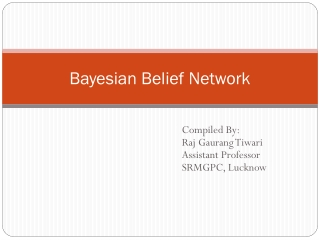DownloadDownload PresentationBayesian Belief Network

# Bayesian Belief Network

Télécharger la présentation## Bayesian Belief Network

- - - - - - - - - - - - - - - - - - - - - - - - - - - E N D - - - - - - - - - - - - - - - - - - - - - - - - - - -
##### Presentation Transcript

1. Bayesian Belief Network Compiled By: Raj GaurangTiwari Assistant Professor SRMGPC, Lucknow

2. Bayesian Belief Network • Bayesian belief networks are powerful tools for modeling causes and effects in a wide variety of domains. • They are compact networks of probabilities that capture the probabilistic relationship between variables, as well as historical information about their relationships. • These networks also offer consistent semantics for representing causes and effects

3. Bayesian Belief Networks A (directed acyclic) graphical model of causal relationships Represents dependency among the variables Gives a specification of joint probability distribution Y Z P • Nodes: random variables • Links: dependency • X and Y are the parents of Z, and Y is the parent of P • No dependency between Z and P • Has no loops/cycles X 3

4. Bayesian Belief Network • Each of the variables in the Bayesian belief network are represented by nodes. • Each node has states, or a set of probable values for each variable. Nodes are connected to show causality with an arrow indicating the direction of influence. These arrows are called edges.

5. Example • A belief network consists of nodes (labeled with uppercase bold letters) and their associated discrete states (in lowercase). Thus node A has states {a1, a2, ...}, which collectively are denoted simply a; node B has states {b1, b2, ...}, denoted b, and so forth. The links between nodes represent direct causal influence. For example the link from A to D represents the direct influence of A upon D. In this network, the variables at B may influence those at D, but only indirectly through their effect on C. Simple probabilities are denoted P(a) and P(b), and conditional probabilities P(c|b), P(d|a, c) and P(e|d).

6. Conditional probability tables • Every node also has a conditional probability table, or CPT, associated with it. • Conditional probabilities represent likelihoods based on prior information or past experience. • A conditional probability is stated mathematically as , i.e. the probability of variable X in state x given parent P1 in state p1, parent P2 in state p2, ..., and parent Pn in state pn. • That is, for each parent and each possible state of that parent, there is a row in the CPT that describes the likelihood that the child node will be in some state.

7. Probability Review Variables and Distributions • Let  a be a discrete variable with N states: • a = { a1, a2, ..., aN } • Then P(a) is the probability distribution of a. • P(a) = {P(a1), P(a2), ... P(aN)} • Where P(a1) + ... + P(aN) = 1

8. Probability Review • Joint Events If  a = { a1, a2, a3 } and b = { b1, b2, b3 } then P(a,b) is the joint probability distribution of a and b. • P(a,b) = {P(a1,b1), P(a1,b2), ..., P(a3,b3) } • where P(ai,bj) is the probability of event ai and bj

9. Probability Review • Chain Rule • We know that P( a,b) = P(a|b) P(b) • This can be expanded to three or more variables: • P(a,b,c) = P(a|b,c) P(b,c) = P(a|b,c) P(b|c) P(c) • P(a,b,c,d) = P(a|b,c,d) P(b|c,d) P(c|d) P(d) • This is known as the chain rule.

10. Belief Network for Fish B1: North Atlantic B2: South Atlantic A1: Winter A2: Spring A3:Summer A4:Autumn A Season B Location X Fish X1: Salmon X2: Sea Bass C Lightness C1: Light C2:Medium C3:Dark D Thickness D1: Wide D2: Thin

11. Example for instance the probability that the fish was caught in the summer in the north Atlantic and is a sea bass that is dark and thin: P (a3, b1, x2, c3, d2) = P (a3)P(b1)P(x2|a3, b1)P(c3|x2)P(d2|x2) = 0.25 × 0.6 × 0.4 × 0.5 × 0.4 = 0.012.